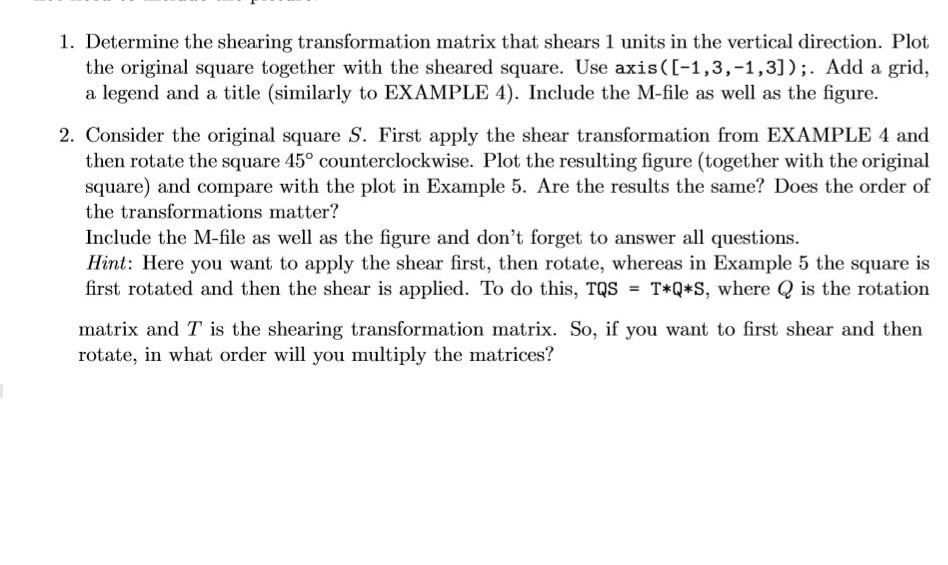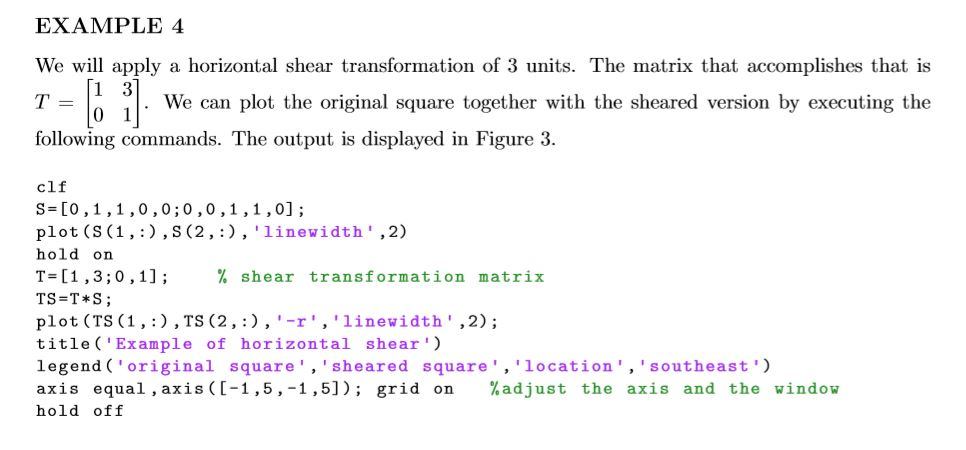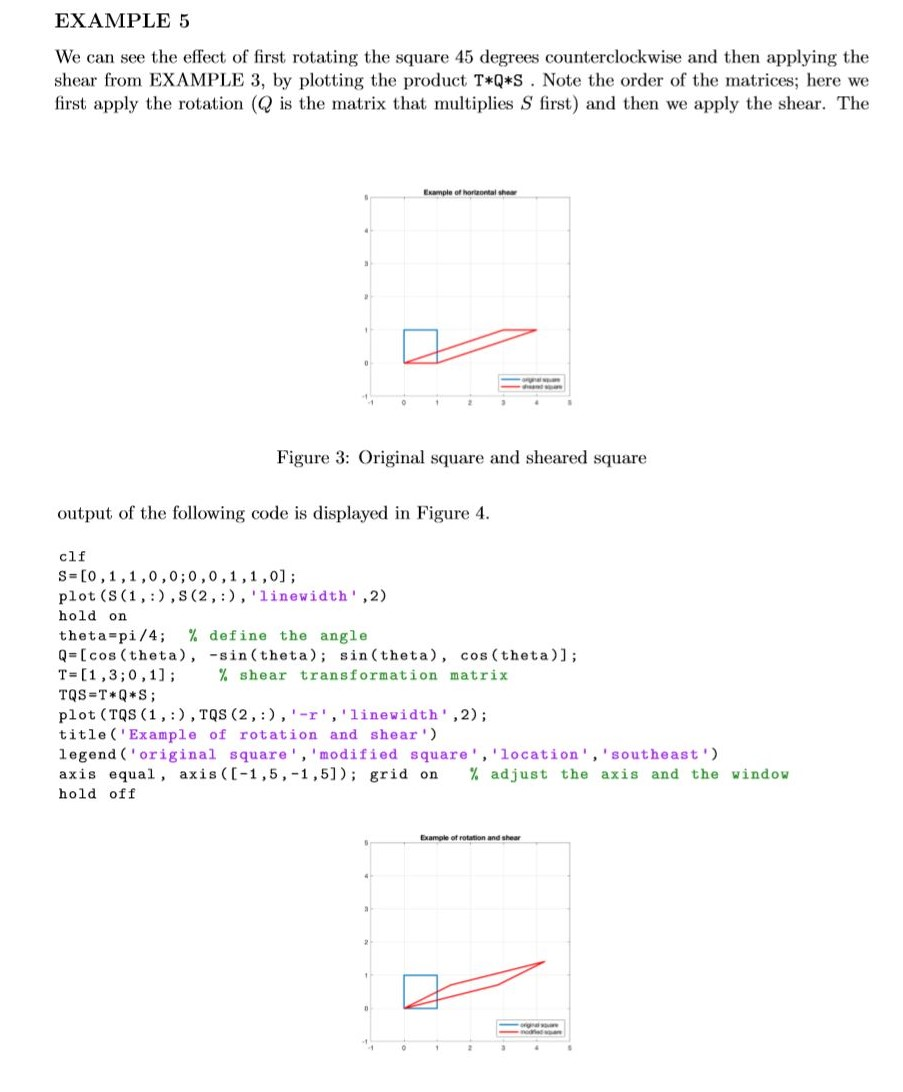# Question *** LOOK AT THE M FILES IN THE EXAMPLES AND THEN MODIFY THEM FOR QUESTION 1 AND 2!!! *** NEEDS TO BE DONE IN MATLAB *** *** NOT AN INCOMPLETE QUESTION *** Look at the examples and modify them for each problem! its not that hard to understand Instructions: For each of the following exercises, create an M-ﬁle to store the MATLAB commands. Copy and paste the M-ﬁle into a text document. For problems 1 and 2, include in the text document the pictures produced by MATLAB. Resize and crop the pictures so that they do not take up too much space. If the question requires written answers, include them in the text ﬁle in the appropriate location. Make sure you clearly label and separate all the Exercises. For problems 3 and 4 you do not need to include the picture. 1. Determine the shearing transformation matrix that shears 1 units in the vertical direction. Plot the original square together with the sheared square. Use axis((-1,3,-1,3]);. Add a grid, a legend and a title (similarly to EXAMPLE 4). Include the M-file as well as the figure. 2. Consider the original square S. First apply the shear transformation from EXAMPLE 4 and then rotate the square 45° counterclockwise. Plot the resulting figure (together with the original square) and compare with the plot in Example 5. Are the results the same? Does the order of the transformations matter? Include the M-file as well as the figure and don't forget to answer all questions. Hint: Here you want to apply the shear first, then rotate, whereas in Example 5 the square is first rotated and then the shear is applied. To do this, TQS = T*Q*S, where Q is the rotation matrix and T is the shearing transformation matrix. So, if you want to first shear and then rotate, in what order will you multiply the matrices? EXAMPLE 4 We will apply a horizontal shear transformation of 3 units. The matrix that accomplishes that is T = We can plot the original square together with the sheared version by executing the 0 following commands. The output is displayed in Figure 3. clf S=[0,1,1,0,0,0,0,1,1,0]; plot (S(1, :), S(2,:), 'linewidth', 2) hold on T=(1,3,0,1); % shear transformation matrix TS=T *S; plot (TS (1, :), TS (2,:),'-r', 'linewidth', 2); title('Example of horizontal shear') legend ('original square', 'sheared square', 'location', 'southeast') axis equal , axis ([-1,5,-1,5]); grid on %adjust the axis and the window hold off EXAMPLE 5 We can see the effect of first rotating the square 45 degrees counterclockwise and then applying the shear from EXAMPLE 3, by plotting the product T*Q*S . Note the order of the matrices; here we first apply the rotation (Q is the matrix that multiplies S first) and then we apply the shear. The Example of horontal show Figure 3: Original square and sheared square output of the following code is displayed in Figure 4. clf S=[0,1,1,0,0,0,0,1,1,0]; plot (S(1,:),S(2,:), 'linewidth', 2) hold on theta-pi/4; % define the angle Q=[cos (theta), -sin(theta); sin(theta), cos (theta)]; T= [1, 3;0,1); % shear transformation matrix TQS=T*Q*S; plot (TQS (1, :), TQS (2,:),'-r', 'linewidth', 2); title('Example of rotation and shear') legend ('original square','modified square', 'location', 'southeast') axis equal, axis ([-1,5,-1,5]); grid on % adjust the axis and the window hold off Example of rotation and shear*** LOOK AT THE M FILES IN THE EXAMPLES AND THEN MODIFY THEM FOR QUESTION 1 AND 2!!!

*** NEEDS TO BE DONE IN MATLAB ***

*** NOT AN INCOMPLETE QUESTION ***

Look at the examples and modify them for each problem! its not that hard to understand

Instructions: For each of the following exercises, create an M-ﬁle to store the MATLAB commands. Copy and paste the M-ﬁle into a text document. For problems 1 and 2, include in the text document the pictures produced by MATLAB. Resize and crop the pictures so that they do not take up too much space. If the question requires written answers, include them in the text ﬁle in the appropriate location. Make sure you clearly label and separate all the Exercises. For problems 3 and 4 you do not need to include the picture.Transcribed Image Text: 1. Determine the shearing transformation matrix that shears 1 units in the vertical direction. Plot the original square together with the sheared square. Use axis((-1,3,-1,3]);. Add a grid, a legend and a title (similarly to EXAMPLE 4). Include the M-file as well as the figure. 2. Consider the original square S. First apply the shear transformation from EXAMPLE 4 and then rotate the square 45° counterclockwise. Plot the resulting figure (together with the original square) and compare with the plot in Example 5. Are the results the same? Does the order of the transformations matter? Include the M-file as well as the figure and don't forget to answer all questions. Hint: Here you want to apply the shear first, then rotate, whereas in Example 5 the square is first rotated and then the shear is applied. To do this, TQS = T*Q*S, where Q is the rotation matrix and T is the shearing transformation matrix. So, if you want to first shear and then rotate, in what order will you multiply the matrices? EXAMPLE 4 We will apply a horizontal shear transformation of 3 units. The matrix that accomplishes that is T = We can plot the original square together with the sheared version by executing the 0 following commands. The output is displayed in Figure 3. clf S=[0,1,1,0,0,0,0,1,1,0]; plot (S(1, :), S(2,:), 'linewidth', 2) hold on T=(1,3,0,1); % shear transformation matrix TS=T *S; plot (TS (1, :), TS (2,:),'-r', 'linewidth', 2); title('Example of horizontal shear') legend ('original square', 'sheared square', 'location', 'southeast') axis equal , axis ([-1,5,-1,5]); grid on %adjust the axis and the window hold off EXAMPLE 5 We can see the effect of first rotating the square 45 degrees counterclockwise and then applying the shear from EXAMPLE 3, by plotting the product T*Q*S . Note the order of the matrices; here we first apply the rotation (Q is the matrix that multiplies S first) and then we apply the shear. The Example of horontal show Figure 3: Original square and sheared square output of the following code is displayed in Figure 4. clf S=[0,1,1,0,0,0,0,1,1,0]; plot (S(1,:),S(2,:), 'linewidth', 2) hold on theta-pi/4; % define the angle Q=[cos (theta), -sin(theta); sin(theta), cos (theta)]; T= [1, 3;0,1); % shear transformation matrix TQS=T*Q*S; plot (TQS (1, :), TQS (2,:),'-r', 'linewidth', 2); title('Example of rotation and shear') legend ('original square','modified square', 'location', 'southeast') axis equal, axis ([-1,5,-1,5]); grid on % adjust the axis and the window hold off Example of rotation and shear
More
Transcribed Image Text: 1. Determine the shearing transformation matrix that shears 1 units in the vertical direction. Plot the original square together with the sheared square. Use axis((-1,3,-1,3]);. Add a grid, a legend and a title (similarly to EXAMPLE 4). Include the M-file as well as the figure. 2. Consider the original square S. First apply the shear transformation from EXAMPLE 4 and then rotate the square 45° counterclockwise. Plot the resulting figure (together with the original square) and compare with the plot in Example 5. Are the results the same? Does the order of the transformations matter? Include the M-file as well as the figure and don't forget to answer all questions. Hint: Here you want to apply the shear first, then rotate, whereas in Example 5 the square is first rotated and then the shear is applied. To do this, TQS = T*Q*S, where Q is the rotation matrix and T is the shearing transformation matrix. So, if you want to first shear and then rotate, in what order will you multiply the matrices? EXAMPLE 4 We will apply a horizontal shear transformation of 3 units. The matrix that accomplishes that is T = We can plot the original square together with the sheared version by executing the 0 following commands. The output is displayed in Figure 3. clf S=[0,1,1,0,0,0,0,1,1,0]; plot (S(1, :), S(2,:), 'linewidth', 2) hold on T=(1,3,0,1); % shear transformation matrix TS=T *S; plot (TS (1, :), TS (2,:),'-r', 'linewidth', 2); title('Example of horizontal shear') legend ('original square', 'sheared square', 'location', 'southeast') axis equal , axis ([-1,5,-1,5]); grid on %adjust the axis and the window hold off EXAMPLE 5 We can see the effect of first rotating the square 45 degrees counterclockwise and then applying the shear from EXAMPLE 3, by plotting the product T*Q*S . Note the order of the matrices; here we first apply the rotation (Q is the matrix that multiplies S first) and then we apply the shear. The Example of horontal show Figure 3: Original square and sheared square output of the following code is displayed in Figure 4. clf S=[0,1,1,0,0,0,0,1,1,0]; plot (S(1,:),S(2,:), 'linewidth', 2) hold on theta-pi/4; % define the angle Q=[cos (theta), -sin(theta); sin(theta), cos (theta)]; T= [1, 3;0,1); % shear transformation matrix TQS=T*Q*S; plot (TQS (1, :), TQS (2,:),'-r', 'linewidth', 2); title('Example of rotation and shear') legend ('original square','modified square', 'location', 'southeast') axis equal, axis ([-1,5,-1,5]); grid on % adjust the axis and the window hold off Example of rotation and shear